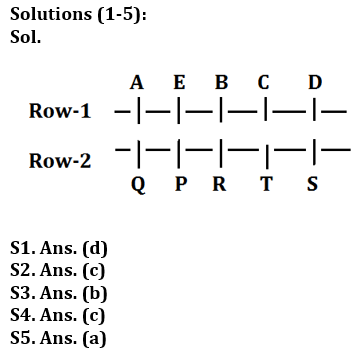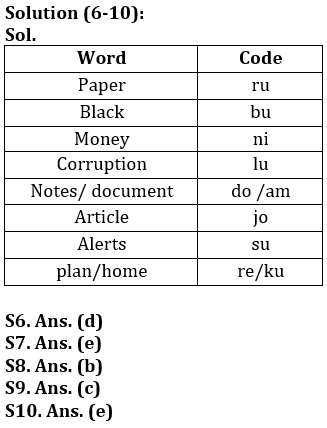Latest Banking jobs   »

# Reasoning Quiz For RBI Grade B Phase 1 2023-25th March

Directions (1-5): Study the information carefully and answer the questions given below.
Ten persons sit in two parallel rows containing five persons in each row such a way that there is an equal distance between adjacent persons. In row-1, A, B, C, D and E are seated and all of them are facing south. In row-2, P, Q, R, S and T are seated and all of them are facing north. Therefore, in the given seating arrangement, each member seated in a row faces another member of the other row.
T sits third to the right of Q. The persons facing P sits to the immediate right of B. Only one person sits between B and D. P sits second to the left of T. A is not an immediate neighbor of B. Only two people sit between A and C. Neither B nor A faces S.

Q1. Who among the following person facing D?
(a) P
(b) Q
(c) R
(d) S
(e) T

Q2. Who among the following sits immediate right of R?
(a) P
(b) S
(c) T
(d) Q
(e) No one

Q3. Who among the following faces the one who sits second to the left of E?
(a) P
(b) T
(c) A
(d) Q
(e) S

Q4. Who among the following sits immediate right of A?
(a) E
(b) B
(c) No one
(d) C
(e) D

Q5. Who among the following sits exactly between A and the one who faces R?
(a) E
(b) C
(c) D
(d) B
(e) None of these

Directions (6-10): Study the following information carefully and answer the questions given below:
In a certain code language
‘Paper home plan black’ is coded as ‘ru ku bu re’
‘Black money alerts’ is coded as ‘ni bu su’
‘Corruption paper money article’ is coded as ‘lu ni ru jo’
‘Notes corruption document’ is coded as ‘do lu am’

Q6. What may be the code for ‘notes money’?
(a) ru ni
(b) do ru
(c) su bu
(d) do ni
(e) Either (b) or (c)

Q7. What is the code for ‘home’?
(a) re
(b) su
(c) ku
(d) lu
(e) Either (a) or (c)

Q8. ‘lu’ is denoted as?
(a) black
(b) corruption
(c) paper
(d) article
(e) None of these

Q9. What is the code for ‘black paper’?
(a) ru ni
(b) bu lu
(c) ru bu
(d) Either (a) or (b)
(e) Cannot be determined

Q10. What may be the code for ‘white plan’?
(a) re dr
(b) ku su
(c) do re
(d) dr ku
(e) Either (a) or (d)

Directions (11-12): Read the following information carefully and answer the given questions.
D, L, N, X, W and V are six friends. Each of them has different weight. L is heavier than only N. W is lighter than only two people. V is not the heaviest. The difference between the weight of third lightest and the lightest person is 12kg. D is heavier than W but is not the heaviest. The weight of V is 70 kg.

Q11. How many persons are heavier than V?
(a) Four
(b) Three
(c) Two
(d) One
(e) None of these

Q12. Which of the following statement is true if weight of L is 66 kg?
(a) The difference between the weight of V and L is 6 kg.
(b) Weight of W is 65 kg.
(c) Weight of D is 24 kg.
(d) The difference between the Weight of L and N is 8 kg.
(e) None is true

Q13. Deepak ranked 31st from the bottom and 13th from the top among those who passed an exam. Six boys did not participate in the exam and four failed in it. How many boys were there in the class?
(a) 54
(b) 55
(c) 52
(d) 53
(e) 51

Q14. In a row of boys, Aman is tenth from the right end and Babloo is eighth from the left end. If in this row Babloo is twelfth from the right then what is the position of Aman from the left?
(a) 9
(b) 10
(c) 11
(d) 12
(e) None of these

Q15. Harmaini is 19th from the left end of a row of 37 students and Harry is 16th from the right end in the same row. How many students are there between them in the row?
(a) 1
(b) 3
(c) 5
(d) 4
(e) 2

SolutionsSolutions (11-12):
Sol. X > D > W > V (70kg) > L > N (58)
S11. Ans. (b)
S12. Ans. (d)

S13. Ans. (d)
Sol. Number of boys who passed = (31 + 13 – 1) = 43
∴ Total number of boys in the class = (43 + 6 + 4) = 53

S14. Ans. (b)
Sol. Clearly, Aman is 10th from the right end and
Babloo is 8th from the left end and 12th from the right end of the row
So, number of boys in the row = (8 -1 + 12) = 19
Now, Aman is 10th from the right
Aman from left end= 19- (10-1)
Hence, Aman is 10th from the left end of the row.

S15. Ans. (e)
Sol. Harmaini position from right end = (37+1-19) = 19
Students between them = (19-16-1) = 2## FAQs

### What is the selection process of the Bank Clerk?

There are 4 sections in the RBI Grade B Phase 1 Exam i.e. English Language, General Awareness, Quantitative Aptitude & Reasoning.

#### Congratulations!Union Budget 2023-24: Free PDF